### Confidence Intervals

#### Parameter Confidence Intervals

The parameter confidence intervals are computed using the Wald-based formula: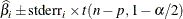where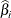is the ith parameter estimate,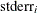is its estimated approximate standard error,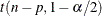is a t statistic with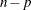degrees of freedom, n is the number of observations, and p is the number of parameters. You can specify the significance levelused in the construction of these confidence limits with the ALPHA= option in the PROC NLIN statement; the default value is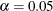. Because Wald-based confidence intervals are only asymptotically valid, you should use confidence curves for a more accurate and complete inference on confidence intervals. See the section Profiling Parameters and Assessing the Influence of Observations on Parameter Estimates for details.

#### Model Confidence Intervals

Model confidence intervals are output when an OUT= data set is specified and one or more of the keywords LCLM, UCLM, LCL, UCL, L95M=, U95M=, L95=, and U95= is specified. The expressions for these terms are as follows: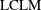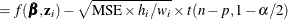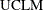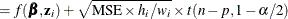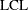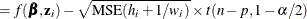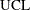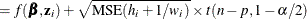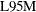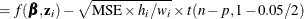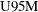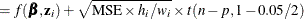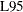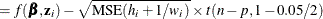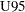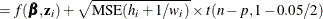where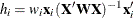is the leverage,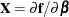, and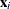is the ith row of. These results are derived for linear systems. The intervals are approximate for nonlinear models. The valuein the preceding formulas for LCLM, UCLM, LCL, and UCL can be set with the ALPHA= option in the PROC NLIN statement or with the ALPHA= option in the OUTPUT statement. If both ALPHA= options are specified, the option in the OUTPUT takes precedence.